U.S. Department of Transportation
1200 New Jersey Avenue, SE
Washington, DC 20590
202-366-4000

Federal Highway Administration Research and Technology
Coordinating, Developing, and Delivering Highway Transportation InnovationsThis report is an archived publication and may contain dated technical, contact, and link information
 Federal Highway Administration > Publications > Research Publications > 06115 > Index, Structural Behavior of Ultra-High Performance Concrete Prestressed I-Girders
Publication Number: FHWA-HRT-06-115
Date: August 2006

# CHAPTER 6. DISCUSSION OF RESULTS

## 6.1 Local and Global Mechanical Failure Modes of UHPC

UHPC exhibits a number of macrostructural mechanical failure modes. These failure modes can be categorized into three specific types: Compressive, tensile cracking, and tensile fiber pullout. A fourth failure mode, the high-cycle fatigue failure of crack-bridging steel fibers, has been observed under special loading conditions but will not be discussed in this report.

The compressive failure of UHPC can be considered to be similar to the compressive failure of any fiber-reinforced concrete. In general terms, UHPC fails under axial compressive load through lateral tensile expansion. This lateral expansion is partially restrained by the internal steel fiber reinforcement, thus allowing for a more ductile failure than may normally be expected. As with any concrete, higher strength UHPC tends to fail in a more brittle manner than lower strength UHPC.

On a large scale, the compressive failure of a girder web being subjected to shear loading was observed during the Girder 28S test. This compression failure can be described as a slow crushing and surface cracking of the UHPC girder web as loads were being redistributed around the failing area. No significant spalling of the concrete was observed during this compression failure. This slow crushing continued for more than 1 minute before the newly redistributed loads caused a tensile fiber pullout failure to occur in another location.

On a smaller scale, compression failures in hundreds of UHPC cylinders were observed while performing ASTM C39 compression tests on cylinders and cores in this study and in the associated material characterization study.(2) The failure of any cylinder that underwent steam-based treatment was brittle, with a rapid load decrease occurring immediately after the peak load was achieved. Even as such, these cylinders remained largely intact with relatively few small fragments leaving the cylinder.

The tensile behavior of UHPC allows for continuing tensile load-carrying capacity across a cracked plane. The design of the structure will determine whether the tensile cracking of UHPC constitutes a failure mode. Further discussion of the tensile behaviors of UHPC as determined from small-scale testing is provided in the associated material characterization report.(2)

The full-scale flexure and shear girder tests provide a significant amount of information regarding the tensile cracking behavior and tensile fiber pullout behavior of UHPC under structural loading. Observations during these tests indicate that tensile cracks form perpendicular to highly stressed tensile regions and their formation is clearly indicated by an audible signal. These cracks were highly linear because the girders contained no passive reinforcement and the UHPC contained no coarse aggregate. The initial cracks that formed in these tests were extremely tight. The only method that could determine the location of these surface cracks was the use of a volatile indicating spray. As the tests progressed, the cracks began to widen slightly and additional cracks filled in nearby. Observations from the full-scale flexure test indicate that crack spacing of 3 mm (0.125 inch) or less is possible in highly stressed regions. These results illustrate UHPC's ability to redistribute stresses and to undergo multiple cracking before fiber pullout.

Final tensile failure of UHPC generally occurs when the steel fiber reinforcement begins to debond from and to pull out of the UHPC matrix. Because fibers are randomly distributed and oriented in the UHPC, individual fiber loads vary at any particular global load level. Mechanically, pullout occurs when the load carried by an individual fiber overcomes the ability of the UHPC to grip the fiber. Pullout by any fibers increases the load that other nearby fibers must carry. Multiple pullouts in a specific location that require gross load redistribution through alternate load paths can be defined as fiber pullout failure.

The full-scale flexure test on Girder 80F and the shear test on Girder 24S provide clear indications of the effect of fiber pullout on global girder behavior. In Girder 80F, hundreds of tightly spaced cracks carried significant flexural tensile forces in the bottom bulb of the girder. At the peak load carried by the girder, the fibers at one specific cross section began to pull out. This crack quickly became significantly wider than any other crack in the girder and placed large additional strains on the prestressing strands at this location. Because the strands could not sustain this large strain, the strands ruptured and the girder failed.

In Girder 24S, many tightly spaced cracks formed perpendicular to the diagonal tensile forces being carried by the web of the girder. As the loads on the girder increased, more cracks formed and the fibers bridging the existing cracks became more highly stressed. Eventually, the fibers bridging a very highly stressed crack began to pull out. Because this girder contained no mild steel reinforcement to aid in load redistribution, the girder abruptly failed.

In both girder tests discussed above, the failure of the girder as a whole was precipitated by the local bond failure between the fibers and the UHPC matrix. These results indicate that unless significant mild or prestressing steel redundancy exists in the UHPC member, fiber pullout will result in member failure.

## 6.2 Development Length of Prestressing Strand in UHPC

Results from two of the UHPC girder shear tests provide an indication of the development length of 1,860 MPa (270 ksi) low-relaxation prestressing strand in this type of concrete. In both tests, sufficient stresses were placed onto prestressing strands with 1.2 m (4 ft) or less of embedment length to cause the strands to rupture.

Section 5.2.1 presents the results from the testing of Girder 28S. Recall that this three-point-loading test setup included a 8.54 m (28 ft) total span, a 2.0 m (6.5 ft) shear span, and a 1.2 m (4 ft) overhang beyond the shear span. This overhanging portion of the girder allowed for the partial to full development of the prestressing strands outside of the region of the girder that traditionally encounters high stresses from flexure or shear. Figure 30 shows the distress that was apparent in this girder at failure. As mentioned in chapter 5, the failure of this girder included the simultaneous rupturing of the two strands in the top flange of the girder directly over the bearing. Because no slip was observed in the instrumented top strand before rupture, it is clear that the full development length of these strands was less than the 1.2 m (48 inches) of embedment between the rupture location and the end of the girder.

The failure of Girder 14S provides further information regarding the development length of this prestressing strand in UHPC. Recall that this three-point-loading test setup included a 4.3 m (14 ft) total span, a 1.8 m (6 ft) shear span, and no overhang (i.e., the bearing was centered 152 mm (6 inches) from the end of the girder). As with all of the girders, half of the strands in the bottom flange were debonded for 0.9 m (3 ft) from the end of the girder. The sequential rupture of the 12 fully bonded strands in the bottom flange defined the failure of this girder. Figure 50 illustrates the distress apparent in the girder at failure, with the strand ruptures occurring at the second large bottom flange crack from the right end of the girder. Note that this crack was 0.94 m (37 inches) from the east end of the girder at the precise location where the full complement of strands became bonded.

Figure 4 shows the strand slip behavior for five of the fully bonded strands in the bottom flange and for five of the debonded strands in the bottom flange, along with one of the top flange strands. The debonded strands all behaved very similarly, showing initial slip at an applied girder load of 2,200 kN (500 kips), 2.5 mm (0.1 inch) of slip at the peak girder load of 3,410 kN (766 kips), and between 8.9 and 12.7 mm (0.35 and 0.5 inch) of slip when the fully bonded strands began to rupture. Of the five fully bonded strands that were monitored, the two in the bottom row of strands showed no slippage before rupture. The three in the second and third rows from the bottom of the girder showed less that 1.5 mm (0.06 inch) of slippage, with the slippage beginning at the girder's peak load capacity and ceasing when these strands ruptured. The results from these five instrumented strands indicate that the development length of UHPC under realistic shear loading conditions is less than 0.94 m (37 inches).

The girder test results discussed above indicate that the development length of 12.7-mm,

1,860-MPa (0.5-inch 270-ksi) low-relaxation prestressing strand in UHPC under realistic full-scale loading conditions is less than 0.94 m (37 inches). In total, one monitored strand ruptured prior to slipping while being subjected to a flexural tensile loading with 1.2 m (48 inches) of embedment, two monitored strands ruptured prior to slipping while spanning 0.94 m (37 inches) through a heavily distressed shear region, and three strands ruptured after a slight slippage while spanning 0.94 m (37 inches) through a heavily distressed shear region. This result is consistent with the findings of Steinberg and Lubbers as discussed in section 2.4.1.(10)

## 6.3 Estimation of Prestress Losses in UHPC Girders

The loss of prestressing force due to instantaneous and long-term effects is an inherent part of pretensioning concrete. In prestressed concrete, the primary instantaneous effect is elastic shortening of the concrete member. The long-term effects comprise concrete shrinkage, concrete creep, and relaxation of the prestressing strand.

This section focuses on determining the prestress losses that occurred in the AASHTO Type II girders. The experimental results for these girders were presented in chapter 5. The AASHTO Load and Resistance Factor Design (LRFD) Bridge Design Specification defines the total loss of prestress as comprising the four effects mentioned above.(19) The specification provides empirical relationships that can be used to estimate these losses. However, these estimations are based on conventional concrete technology; thus, more accurate estimations of UHPC girder behavior can be achieved through the use of the experimental results presented in the associated UHPC material characterization report.(2)

The AASHTO Type II girders discussed in chapters 4 and 5 were each prestressed with 24 strands in the bottom bulb and 2 strands in the top bulb. The fabricator stressed these 1,860-MPa (270-ksi) low-relaxation strands to 55 percent of their ultimate strength, or 1,030 MPa (149 ksi). The strand stressing was completed within 1 day before the casting of the UHPC. After casting, approximately 3 days passed before each girder was stressed. The girders then were steam treated.

The time-dependent prestress loss due to shrinkage of the UHPC can be approximated based on the experimental results determined in the associated UHPC material characterization study.(2) The early age unrestrained shrinkage of UHPC was determined to be approximately 500 microstrain at 3 days after casting. The final stabilized asymptotic shrinkage value after steam treatment was determined to be approximately 850 microstrain. Thus, 350 microstrain of shrinkage can be expected to occur after the stressing of the girder. The strands can be assumed to have a stiffness of 197 GPa (28,500 ksi); therefore, this shrinkage will reduce the stress in the strand by 69 MPa (10.0 ksi).

The time-dependent prestress loss due to creep of the UHPC also can be approximated based on the experimental results determined in the associated UHPC material characterization study.(2) An elastic analysis of the UHPC girders at transfer indicates that the stress in the concrete at the centroid of the prestress is approximately 21 MPa (3.0 ksi). The strength of the concrete at transfer would have been at least 69 MPa (10 ksi) and would have continued to increase both before and during steaming until the final compressive strength of 193 MPa (28 ksi) was achieved. Based on this information, the level of stress on the concrete was clearly at or below 30 percent of the compressive strength.

The creep coefficient for UHPC can vary depending on the age of the concrete and the curing treatment that has been applied. The associated UHPC material characterization study(2) found that a creep coefficient of 0.3 can be expected after the steam treatment, but that the creep coefficient could be as high as 0.78 if untreated UHPC is subjected to a compressive stress equal to 67 percent of its compressive strength. Based on these results, a reasonably conservative aggregate value for the long-term creep coefficient of UHPC stressed as described above is estimated to be 0.5.

Additionally, this analysis requires an estimation of the modulus of elasticity of the UHPC throughout the timeframe during which creep is occurring. Experimental results have indicated that the modulus of elasticity can range from 42.7 to 52.7 GPa (6,200 to 7,650 ksi) for the 28-day untreated to steam-treated UHPC, respectively.(2) Also, early age modulus of elasticity testing has indicated that the modulus of elasticity for 69 MPa (10 ksi) UHPC will be approximately 34.5 GPa (5,000 ksi). Conservatively, the modulus of elasticity for this analysis is assumed to be 41.4 GPa (6,000 ksi) because a smaller modulus will result in larger overall creep losses.

The goal of this creep analysis is to determine the stress loss in the strands resulting from the creep strain imparted into the concrete. The creep strain in the concrete is calculated by multiplying the creep coefficient by the initial concrete strain at prestress transfer. This concrete strain can be approximated to equal the 21 MPa (3.0 ksi) initial prestress divided by the modulus of elasticity of 41.4 GPa (6,000 ksi). Thus, the creep strain in the concrete is 240 microstrain, and the resulting loss of prestress in the strands is 48 MPa (6.9 ksi).

The elastic shortening loss in the UHPC girder was calculated based on a strain compatibility analysis of the girder cross section. All prestressing strands were considered separately; thus, strands at different distances from the neutral axis exhibited different levels of stress. The modulus of elasticity of the UHPC was assumed to equal 34.5 GPa (5,000 ksi) at transfer. The elastic shortening of the UHPC girder at the center of gravity of the prestressing strands is 540 microstrain. Multiplying this value by the modulus of elasticity of the strands results in an average elastic shortening loss in the strands of 106 MPa (15.4 ksi).

Finally, the time-dependent losses due to strand relaxation after transfer were calculated based on the relationship provided in the AASHTO LRFD Bridge Design Specifications.(19) The calculation estimates the relaxation loss by relating it to the other three losses detailed above. Specifically, this loss equals 41 MPa (6 ksi) minus 12 percent of the elastic shortening loss minus 6 percent of the creep and shrinkage losses. In total, the strand relaxation loss for the UHPC girder described above is 21.4 MPa (3.1 ksi).

The total prestress losses for this prestressed girder equal 245 MPa (35.6 ksi). This value includes the time-dependent 69 MPa (10.0 ksi) shrinkage loss, the 48 MPa (6.9 ksi) creep loss, and the 21.4 MPa (3.1 ksi) strand relaxation loss. It also includes the 106 MPa (15.4 ksi) elastic shortening loss that occurs at the stressing of the girder. Because the girders were only stressed to 55 percent of the ultimate strand strength, the time-dependent losses total 14 percent of the strand jacking stress and the instantaneous elastic losses total 10 percent of the strand jacking stress.

These losses are of similar magnitude to the losses that normally occur in prestressed concrete girders, which seems to indicate that UHPC provides minimal benefit in terms of prestress loss behaviors. However, it must be noted that the low level of initial jacking stress results in a significantly larger percentage of stress loss than would occur with a higher initial jacking stress. Also, the stressing of the girder when the UHPC compressive strength is 69 MPa (10 ksi) results in much higher losses than would occur if the UHPC had reached 103 MPa (15 ksi) or more without steam treatment or had reached 193 MPa (28 ksi) with steam treatment. These higher compressive strengths would result in decreased shrinkage strains, decreased creep strains, and a higher modulus of elasticity that would reduce elastic shortening.

## 6.4 Flexural Behavior of Prestressed UHPC Girders

The static loading of Girder 80F provided significant information regarding the full-scale flexural behavior of UHPC girders. The results of this test are presented in section 5.1. The following sections provide an indepth analysis of these test results with a focus on modeling the global girder behaviors.

### 6.4.1 Analytical Predictions of Global Behavior

Simple, accepted analytical methods exist for the prediction of the flexural behavior of prestressed concrete girders. These methods are based both on structural mechanics and on empirical relationships. In general, these empirical relationships approximate the nonlinear stress-strain behaviors of normal reinforced concrete. Although these analytical methods cannot accurately model the entire mechanical behavior of UHPC, the flexural capacity and centerline load-deflection behaviors were analyzed using these techniques for comparative purposes.

Recall from previous discussions that Girder 80F is an AASHTO Type II girder spanning 23.9 m (78.5 ft) and composed of 193-MPa (28-ksi) UHPC. The girder is prestressed with twenty-four 12.7-mm, 1860-MPa (0.5-in, 270-ksi) low-relaxation strands in the bottom flange and two strands in the top flange. It contains no mild steel reinforcement. The strands were all initially stressed to 55 percent of their ultimate strength. As discussed in section 6.3, the time-dependent stress losses in the strands total 14 percent.

The initial elastic behavior of the girder was analyzed through a strain compatibility analysis. In this analysis the prestress forces are applied to the girder cross section at discrete locations, thus allowing strands at different depths from the neutral axis to apply different force levels onto the girder. The stiffness of the concrete at girder stressing, Ec,stressing, was approximated to be 34.5 GPa (5,000 ksi) for this portion of the analysis, which determines the elastic shortening losses in the strands. The girder's self-weight flexural stresses also were calculated using this concrete stiffness. The time-dependent losses then were applied to the girder through the use of a second concrete stiffness, Ec,losses. This value was assumed to be 41.4 GPa (6,000 ksi) for Girder 80F.

The preceding analyses determine the state of stress in the girder at the initiation of live load application. Based on these results, the flexural capacity of the girder can be approximated. For normal concrete, this analysis requires only an empirical approximation of the compressive stress-strain behavior. The modeling presented here is based on the assumptions that the Whitney stress block is applicable to UHPC and that UHPC carries no tensile forces after cracking. This second assumption is significantly in error, as will be discussed in section 6.4.5.

The peak load applied to Girder 80F was 790 kN (178 kips), corresponding to an applied moment of 4,370 kN-m (38700 kip-inches) with the neutral axis located 0.25 m (10 inches) down from the top of the girder. A flexural analysis of the girder that was based on the analytical techniques discussed above indicates that the moment capacity should be 3,150 kN-m (27,840 kip-inches) with the neutral axis located 0.13 m (5 inches) down from the top of the girder at failure. An additional flexural analysis was completed to compare the capacity of this girder to an HPC girder of identical configuration with a compressive strength of 55 MPa (8 ksi). The modulus of elasticity of this girder was assumed to be 35.5 GPa (5,150 ksi) based on AASHTO LRFD equation 5.4.2.4-1.(19) The analysis indicates that the flexural capacity of this girder is 2,310 kN-m (20,400 kip-inches) with the neutral axis located 0.42 m (16.5 inches) down from the top of the girder at failure.

Modeling the load-deflection behavior requires further approximations of elastic behavior limits and inelastic behaviors. Specifically, approximations of the tensile cracking stress and the effective flexural stiffness of the cross section are required. AASHTO LRFD equation 5.7.3.6.2-1 was used to model the postcracking flexural stiffness of the girder.(19) The tensile cracking strength, fct, of the UHPC was approximated by the equation for normal weight concrete presented in AASHTO LRFD section 5.4.2.6.(19)

Figure 51 presents the load-deflection response for Girder 80F and for three other cases analyzed through the methods discussed above. One of the alternate cases corresponds to the flexural capacity of the 55-MPa (8-ksi) HPC girder, and the other two alternate cases both correspond to the 193-MPa (28-ksi) UHPC flexural capacity analysis. The tensile cracking strength of the 55-MPa (8-ksi) concrete is assumed to be 4.6 MPa (0.67 ksi). The tensile cracking strength of the 193-MPa (28-ksi) concrete is assumed to be 8.6 MPa (1.25 ksi) in one case and 20 MPa (2.9 ksi) in the other. These tensile strengths represent the AASHTO LRFD compressive strength-based approximation and the observed tensile cracking strength, respectively.(19)

A comparison of the observed girder flexural behavior with the three other curves shown in figure 51 indicates three primary findings. First, it is clear that Girder 80F carried a significant additional load and displayed significant additional ductility as compared with the predicted behavior of a 55-MPa (8-ksi) HPC girder. Second, the significantly decreased flexural capacity and residual stiffness of the two theoretical 193-MPa (28-ksi) girders indicate that the elimination of the fiber reinforcement's contribution to the strength and stiffness approximations results in a very conservative answer. Finally, the difference between the two 193 MPa (28 ksi) girder theoretical approximations indicates that the tensile cracking strength of UHPC has a significant impact on the deflection response of a girder at a particular load level.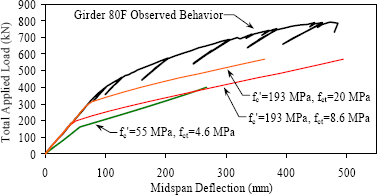1 kN = 0.225 kip
1 MPa = 145 psi
1 mm = 0.039 inch

Figure 51. Graph. Predicted behavior of girders tested in the configuration of Girder 80F.

### 6.4.2 Cracking Behavior

The tensile cracking behavior of prestressed UHPC girders has been observed to be significantly different than would be expected in normal concrete girders. As discussed in section 5.1 and as presented in figure 16, the tensile flange of this prestressed girder displayed a very high crack spacing density. Also, the crack spacing throughout the span seemed proportional to the flexural forces applied. The focus of this section is to derive a general relationship between the cracking behavior of UHPC in the tension flange of a prestressed concrete girder and the strain observed in the girder.

The load and strain data collected during the testing of Girder 80F, along with the crack spacing density observations, allows for the derivation of a relationship between the apparent UHPC strain and the cracking of UHPC. The basic assumption required to initiate this derivation is that the girder is undergoing pure flexural behavior with plane sections remaining plane. Under pure flexural behavior, the neutral axis location and curvature of the midspan cross section recorded during the testing of Girder 80F can be used to derive the effective strain in the bottom flange of the girder at any applied live load moment. This calculation was performed, and the results for each data point recorded throughout the test are presented in figure 52. A curve, also shown in the figure, was then fit through the data to define a continuous relationship between these two variables.

The preceding analysis has allowed for a general relationship between the applied moment and the bottom flange strain. The next step is to determine the actual bottom flange strain in the girder at the load step where the crack spacing observations were recorded. As previously mentioned, the crack spacing observations were recorded when the total applied load on the girder was 690 kN (155 kips), thus defining the applied moment throughout the span and, with figure 52, the bottom flange strain. The preexisting bottom flange strain due to prestress forces and dead load were calculated based on the known prestress forces, the assumed prestress losses, and the dead weight of the girder. Recall that the 14 percent prestress losses resulted in an overall prestressing force of 2,020 kN (455 kips) at an eccentricity of 0.23 m (9.15 inches) below the elastic neutral axis. The addition of the live load strains to the preexisting strains results in the total tensile strain that existed in the bottom flange of the girder at the time of the crack density observations.

The specific crack spacing observations from Girder 80F are presented in figure 53. This figure shows the crack spacing in terms of the distance from midspan. The calculations discussed above allow for the transformation of this distance value into the strain in the UHPC caused by the prestressing forces, dead load, and live load. The results of this transformation are presented in figure 54. This figure, presented in semilog format, displays the observed crack spacing as related to the strain in the UHPC.

Figure 54 also presents a curve representing the best fit equation that was fit to the strain versus crack spacing results. The equation for this curve is presented in figure 55. The crack spacing, w, in millimeters can be determined from the strain, e, in microstrain and the constants a through d that equal 2.87, 1.82E+06, −5.72E+07, and 3.66E+08, respectively.

Alternatively, the crack spacing could be viewed as the independent variable. This revision allows for the estimation of strain based on crack spacing. Figure 56 revises the previous figure into this more convenient format. This figure also graphically presents the equation shown in figure 57. This equation provides a more convenient format for determining the strain in microstrain based on the crack spacing in millimeters. Here, the variables a through c are 450, 500, and 40, respectively.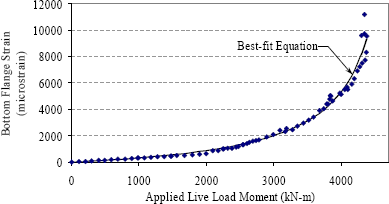1 kN-m = 8.85 kip-inches

Figure 52. Graph. Girder 80F midspan bottom flange strain throughout testing.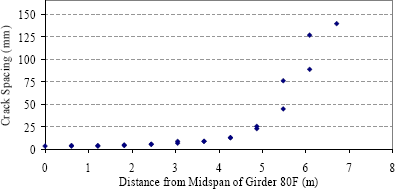1 m = 3.3 ft
1 mm = 0.039 inch

Figure 53. Graph. Flexural crack spacing observed on the bottom flange of Girder 80F at a total applied load of 690 kN (155 kips).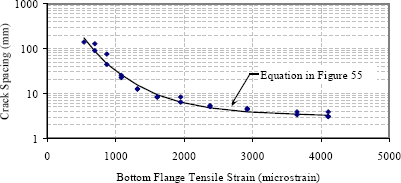1 mm = 0.039 inch

Figure 54. Graph. Flexural crack spacing related to tensile strain.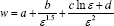Figure 55. Equation. Crack spacing as a function of strain.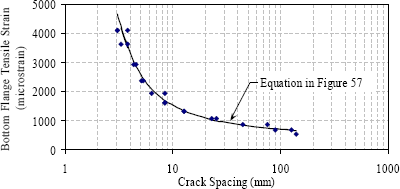1 mm = 0.039 inch

Figure 56. Graph. Tensile strain related to flexural crack spacing.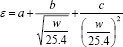Figure 57. Equation. Strain as a function of crack spacing.

### 6.4.3 Effective Moment of Inertia

Elastic flexural analysis was used to determine the stiffness of Girder 80F, including both before and after flexural cracking of the girder. The testing of Girder 80F included periodic partial unloading cycles. During these cycles, approximately 30 percent of the load was removed from the girder before being reapplied incrementally. The data collected during the reloading cycles allow for the analysis of the residual elastic behavior of the girder. This analysis focuses on the load-deflection behavior of the girder.

These slopes were then used to determine the effective moment of inertia, Ieff, of the girder as a whole. A revision of standard flexural deflection relationships allows for the calculation of Ieff through the use of the known geometry of the loading. The equation in figure 58 provides the relationship between the slope of the load deflection response, mP/D, and the load, P, and Ieff for deflections measured at the centerline of the girder. This equation includes variables for the girder span, L, the distance from the bearing to the load point, a, the dead load of the girder, w, and the initial uncracked moment of inertia of the cross section, Iuncracked. This analysis accounts for the effects of the dead load on the residual stiffness of the girder.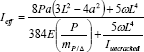Figure 58. Equation. Relationship between the midspan vertical deflection of a girder and its effective moment of inertia.

The equation in figure 59 is very similar to the previous equation, except that it is a generalized relationship that is applicable for deflections recorded at any point on the girder between a bearing and its closest load point. In this equation, x is the distance from the bearing to the location of the deflection measurement.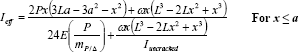Figure 59. Equation. Relationship between the vertical deflection of a girder and its effective moment of inertia.

The analysis described above allows for the determination of Ieff values for the girder at various load levels and at various locations across the span. The results, presented in figure 60, from the six deflection measuring locations were averaged for each load level. The Ieff values are displayed on the midspan load-deflection response of the girder, with Ieff being indicated at the location on the curve for which each analysis was conducted. The value calculated based on the initial loading of the girder is shown in the lower right corner of the figure.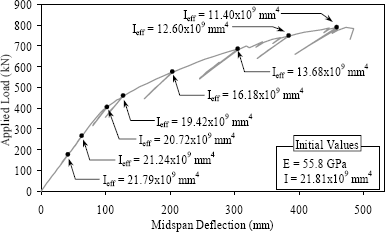1 kN = 0.225 kip
1 mm = 0.039 inch
1 GPa = 145 ksi
1 mm4 = 2.403 x 10-6 in4

Figure 60. Graph. Effective moment of inertia of Girder 80F.

### 6.4.4 Flexural Stiffness Under Flexural Loading

Observations gathered during the testing of Girder 80F and calculations completed after the test indicate that the overall flexural behavior of this girder is more likely to be accurately modeled by standard structural mechanics than would normally be expected for concrete girders. The cracking behavior of UHPC wherein the material continues to possess tensile strength and stiffness after cracking is the driving factor behind this conclusion. Based on the fact that the deflection and rotation of the girder are known at all load levels up to failure, it is reasonable to perform a section-based analysis of the girder wherein the flexural stiffness of the girder at each cross section will be determined based on the overall girder behavior.

A flexural analysis of Girder 80F was performed to determine the flexural stiffness of any cross section along the length of the girder as a function of the live and dead load moment, M, applied to the girder at that location. The virtual work analysis technique was used. The equation in figure 61 provides the basic analytical relationship wherein the moment on the girder, M, is multiplied by the moment, m, generated in the equilibrium system by a "dummy" load at the location where the deflection, D, is desired. The flexural stiffness of the girder, EI, which also varies along the length of the girder, is included as well. The integration, or in this case the summation, of nearly 1,000 discrete cross-sectional slices along the length of the girder provides the solution. Note that shear deformations were excluded from the analysis because they were assumed to be minimal given the loading and girder configurations.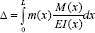Figure 61. Equation. Virtual work relationship between applied moment and deflection.

In this analysis, the UHPC modulus of elasticity was held constant and the moment of inertia was allowed to vary to achieve the correct overall flexural stiffness, K. The initial value for the modulus of elasticity was determined through the method described above with the uncracked moment of inertia of the girder, Iuncr, equal to 21.89x109 mm4 (52,400 inches4). Recall that this value is the moment of inertia as calculated for the transformed section at the time the girder was tested. The initial elastic behavior of the girder as recorded through the deflection and rotation gages indicates that the overall modulus of elasticity of the girder is 55.8 GPa (8,100 ksi). Additionally, recall that the experimentally observed dead plus live load cracking moment of the girder is 20.1 kN-m (17,800 kip-inches), and the corresponding moment capacity was 47.8 kN-m (42,300 kip-inches).

The analysis described above was completed on an iterative basis. First, a potential relationship was proposed between the flexural stiffness and the total moment. The analysis was performed to determine the resulting deflections and rotations at each of the potentiometers and tilt meters. This analysis was repeated for each observational step (or load level) throughout the experimental testing of the girder. The analytical results were then compared with the experimental observations, and the K versus M relationship was revised accordingly. The analyses then were repeated until a sufficiently accurate flexural stiffness was determined.

The equation in figure 62 provides the flexural stiffness relationship that was computed for the AASHTO Type II girder tested in this research. This cumulative, exponentially modified Gaussian equation relates K to M through six variables that define the shape of the curve. Figure 63 graphically presents the relationship. Note how the stiffness of the cross section does not begin to decrease until the moment has increased well above the cracking moment. This behavior is due to the cracking spacing behavior and postcracking load-carrying ability of UHPC as discussed earlier in this chapter.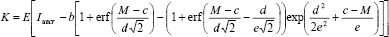with: K = flexural stiffness of girder cross section, kN-m2

M = live plus dead load moment on girder cross section, kN-m.

E = 55.8x106 kN/m2 (i.e., 55.8 GPa)

Iuncr = 0.02181 m4

b = 0.01255 m4

c = 2,343 kN-m

d = 65.9 kN-m

e = 2,588 kN-m

Figure 62. Equation. Cross-sectional flexural stiffness as a function of applied moment.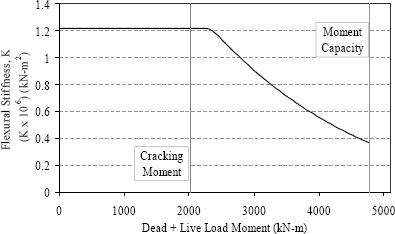1 kN-m = 8.85 kip-inches
1 kN-m2 = 348 kip-inches2

Figure 63. Graph. Flexural stiffness of an AASHTO Type II girder.

Figures 64 through 68 present the results of a comparison between the analytical deflection or rotation calculated using the equation in figure 63 and the experimentally observed behavior. The results of this comparison are displayed for the six monitored deflections and the two rotations for each of the 122 observational steps that comprise the girder test. The mean value of the predicted-to-observed ratio is presented in figure 64. This figure shows that the predicted deflections and rotations are within 5 percent of the experimental values throughout nearly the entire test.

Figures 65 through 68 provide graphical representations of the accuracy of the flexural stiffness model. Figure 65 presents the experimentally observed midspan deflection results and the analytical values. Figure 66 focuses on the deflection results at the load points, and figure 67 focuses on the deflection at the quarter points. Finally, figure 68 presents the experimental and analytical results for the rotation at the ends of the girder. In all cases, note the accuracy with which the analytical model corresponds to the experimentally observed behaviors.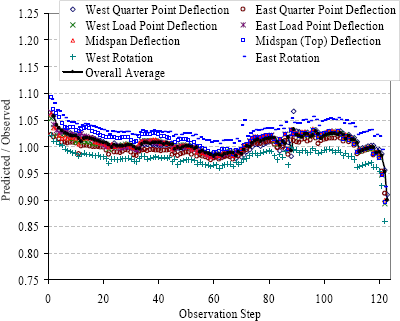Figure 64. Graph. Ratio of predicted deflections and rotations to experimental results.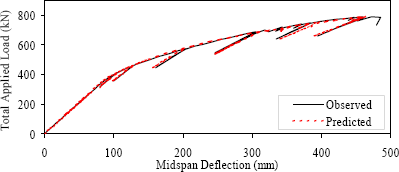1 kN = 0.225 kip

Figure 65. Graph. Predicted and observed midspan deflection results.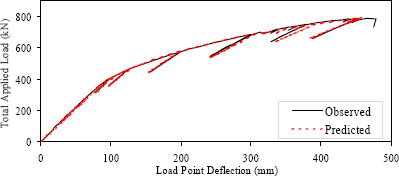1 kN = 0.225 kip
1 mm = 0.039 inch

Figure 66. Graph. Predicted and observed load point deflection results.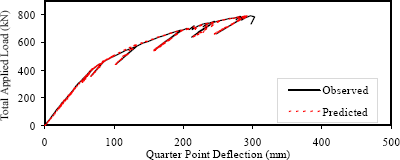1 mm = 0.039 inch
1 kN = 0.225 kip

Figure 67. Graph. Predicted and observed quarter point deflection results.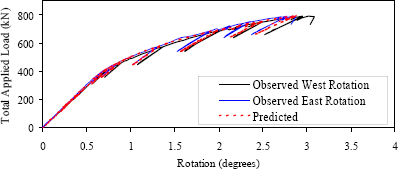1 kN = 0.225 kip

Figure 68. Graph. Predicted and observed end rotation results.

### 6.4.5 Uniaxial Stress-Strain Model of Girder Flexural Behavior

The effective structural use of UHPC requires knowledge of its uniaxial stress-strain behavior. An analytical derivation of the uniaxial stress-strain response of the UHPC was obtained from the experimental results of the flexural testing of Girder 80F. This section presents the basis of this derivation and the derived stress-strain response.

The testing and instrumentation setup for Girder 80F was presented in chapter 4, and the test results were presented in chapter 5. One principal component of these results was the strain profile on the midspan cross section of the girder. This strain profile was determined based on bonded strain gage readings in uncracked regions of the cross section on both faces of the girder. Plane sections are assumed to remain plane in this analysis; therefore, the strain profile results were then extrapolated to determine both the strains at the top and bottom of the cross section and the location of the neutral axis. Figure 69 shows these results for each load step throughout the test. The experimental results also included the moment applied to midspan of the girder throughout the test and the composite stiffness of the girder as derived in section 6.4.4. Finally, an approximation of the compressive stress-strain response of UHPC was derived in the associated UHPC material characterization report.(2)

The state of strain in the girder at the initiation of flexural loading must be known to complete this analysis. Before the application of flexural live load, the only stresses at midspan are caused by the prestress force and the dead load. An analysis was completed to determine the stresses in the UHPC and the prestressing strands. The basic assumptions in this analysis included that plane sections within the cross section remain plane and that strain compatibility between the strands and the concrete is maintained. The prestressing force and dead load were applied to the girder when its compressive strength was at least 69 MPa (10 ksi) and its modulus of elasticity was 34.5 GPa (5,000 ksi). The prestressing losses discussed in section 6.3 then were applied after the modulus of elasticity increased to 41.4 GPa (6,000 ksi). The compressive stresses in the concrete at the initiation of the Girder 80F test were determined to be 6.9 MPa (1.0 ksi) at the top of the midspan cross section and 10.3 MPa (1.5 ksi) at the bottom of the midspan cross section. The stresses in the strands ranged from 842 MPa (122.2 ksi) in the top flange strands to 820 MPa (118.9 ksi) in the lowest bottom flange strands.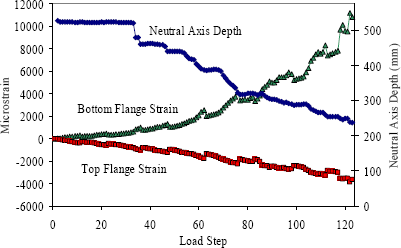1 mm = 0.039 inch

Figure 69. Graph. Experimental strain profile results for midspan of Girder 80F.

These concrete and strand stress values were then transformed into equivalent strain values on the cross section. This transformation assumed linear elastic behavior with 55.8 GPa (8,100 ksi) and 196.5 GPa (28,500 ksi) as the moduli of elasticity for the UHPC and the prestressing strands, respectively. Thus, the linear strain profile on the cross section at the Girder 80F test's initiation included 123 microstrain of compression at the top of the girder and 190 microstrain of compression at the bottom. The strain in the top prestressing strands was 4,287 microstrain, and the four layers of strands in the bottom flange had strains of 4,192; 4,185; 4,178; and 4,170 from top to bottom.

These results provide the basis for the analysis that was completed to determine the full uniaxial stress-strain behavior of UHPC. The analytical procedure included the following steps. First, the midspan cross section of the girder was discretized into 72 concrete slices and 5 prestressing strand slices parallel to the neutral axis. The strain on each of these slices at every load step throughout the test was then calculated based on the observed strain profiles. A complete uniaxial stress-strain response for the UHPC was then assumed. Using this stress-strain response and assuming that the prestressing strands behaved in a linear elastic manner, the stresses on the cross section at each load step were calculated. Note that this analysis initially focused on the loading steps throughout the test and ignored the unloading and reloading steps.

The summation of the stresses in the concrete and the strands then were compared to ensure that the internal forces on the cross section were in equilibrium at every load step. The assumed UHPC uniaxial stress-strain response was repeatedly modified, and the calculations were recomputed until the internal forces on the cross section were sufficiently coincident. Figure 70 shows the uniaxial stress-strain response that was derived through this analytical technique. Figure 71 shows the force in the concrete, the force in the strands, and the ratio of the two values for each load step. On all loading steps the concrete and strand forces are within 7 percent of one another. This result is considered sufficiently accurate, given the experimental variability inherent in the test results that are the basis for these calculations.

Figure 70 is composed of four separate curves. Each curve defines a portion of the behavior. In compression, the UHPC follows the relationship defined in the associated UHPC material characterization report with a modulus of elasticity of 55.8 GPa (8,100 ksi).(2) The initial tensile behavior of UHPC is considered to be linear elastic with an 55.8-GPa (8,100-ksi) modulus of elasticity. The postcracking behavior of UHPC also is shown in figure 70, with an initial modulus of elasticity of 34.5 GPa (5,000 ksi). The final portion of the behavior is the tensile transition zone during the initial cracking of the concrete. This transition allows the UHPC to transform from an uncracked, elastic material into a significantly cracked material that is still capable of carrying tensile loads.

Note that the UHPC has to carry 20.7 MPa (3.0 ksi) of tensile stress before the cross section becomes sufficiently cracked to cause the stress-carrying capacity to decrease. This value is well above the tensile cracking strength that was determined in the UHPC material property characterization testing, because those tests focused on first cracking while the girder test focused on sufficient cracking to change global behaviors. Small-scale tensile tests tend to limit the load path redundancy, thus allowing initial crack(s) to grow longer and wider before other cracks form. Full-scale prestressed girders offer redundancy in two ways. First, the prestressing strands tend to help maintain tight cracks. Second, the large scale of the girder eliminates the localized stiffness effects caused by single cracks on prism flexure specimens.

To complete this analysis, the unloading and reloading behaviors of UHPC were investigated. Similar to most structural materials, after UHPC undergoes inelastic deformation, subsequent unloading and reloading of the concrete causes it to behave in a linear elastic manner with a reduced modulus of elasticity. This continues until new strain levels are attained. In this analysis, UHPC not experiencing peak tensile or compressive strains was assumed to behave in a linear elastic manner with a stiffness equal to the secant modulus of elasticity of the peak strain ever attained. This assumption was used both in tension and compression. For the postcracking tensile behaviors, as shown in figure 70, the origin for the secant modulus was shifted to the location where the red curve is shown to intersect the x-axis. In the tensile cracking transition region, the secant moduli were calculated just before and after the transition region. The secant values in the transition region were then linearly interpolated from these end values.

The results of this analysis are presented in figure 72. This figure shows the experimentally captured external moments that were applied to the midspan cross section. The figure also shows the internal moments on the cross section caused by the assumed stress-strain response at every load step. The results are quite accurate until the final load steps of the test. At this point the model tends to overestimate the internal moment, which is understandable for two reasons. First, the experimentally determined strain profile on the cross section is least accurate at these high loads when the cross section is significantly cracked and the neutral axis has moved closer to the top flange. Second, the method used to determine the uniaxial stress-strain response builds on itself throughout the test. Therefore, more accuracy is built into the lower tensile and compressive strain ranges than can be expected in the higher strain ranges.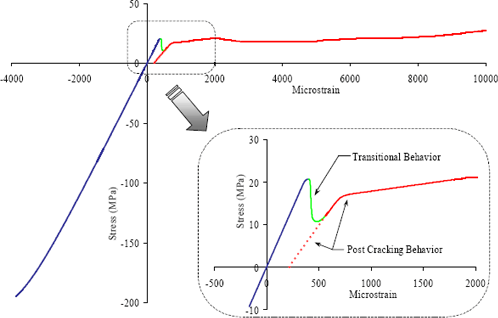1 MPa = 145 psi

Figure 70. Graph. Analytically derived uniaxial stress-strain behavior of UHPC.

The final portion of this analysis focuses on determining the stiffness exhibited by the UHPC throughout its uniaxial behavior. In section 6.4.4, the stiffness of this UHPC AASHTO Type II cross section was determined for the range of applied moments encountered in this test. Thus, the total stiffness of the midspan cross section, as analyzed in this section of the report, should add up to the stiffness determined in section 6.4.4. Figure 73 presents the results from section 6.4.4 and the stiffness results from this analysis. The UHPC stiffness on the loading branch in compression and in tension prior to cracking was defined by the tangent modulus of elasticity. The stiffness was defined by the secant modulus of elasticity during unloadings and reloadings, and after cracking. This method of determining the moment of inertia of the UHPC flexural section provides very accurate results, with only a slight underestimation of the stiffness after cracking has occurred, as shown in figure 73.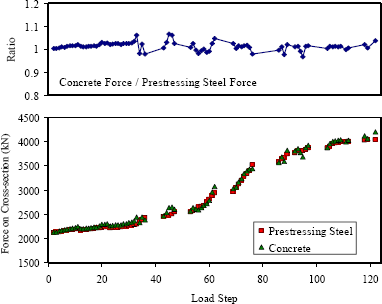1 kN = 0.225 kip

Figure 71. Graph. Summation of forces on cross section during loading steps.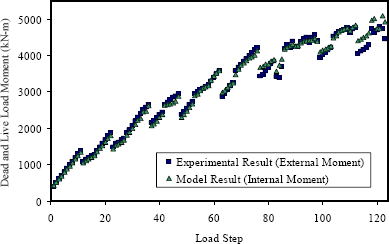1 kN = 0.225 kip

Figure 72. Graph. External and internal moments on midspan cross section.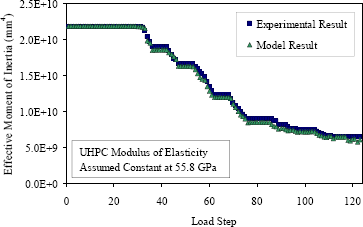1 mm4 = 2.403 x 10-6 in4

Figure 73. Graph. Internally and externally determined moment of inertia.

## 6.5 Shear Behavior of Prestressed UHPC Girders Containing No Mild Steel

Three AASHTO Type II prestressed UHPC girders were failed in shear as part of this research program. The results of these tests were presented in chapter 5. This section combines the results from these three tests. First, the shear capacity results are compared with the predicted capacity calculation results. Second, the shear cracking behavior of UHPC is discussed. The final part of this section focuses on a truss model developed to explain the behaviors observed in one particular shear test.

### 6.5.1 Predicted Versus Actual Global Behavior

The primary goal of the three full-scale shear tests was to determine the shear capacity of prestressed UHPC girders. To summarize the results presented in chapter 5, Girder 28S had a shear capacity of 1,710 kN (384 kips) and failed due to a preexisting horizontal crack at the base of the web. Girder 24S had a shear capacity of 2,230 kN (502 kips) and failed due to diagonal tension in the shear region. Girder 14S had a shear capacity of 1,950 kN (438 kips) and failed due to a combination of diagonal tension and strand slip. These results indicate that the shear capacity of a 0.91-m-deep (36-inch-deep) prestressed concrete girder can be as high as 2,230 kN (500 kips) without any standard shear reinforcement. Under a normal loading condition, in which strand slip might be expected, a shear capacity of more than 1,780 kN (400 kips) still can be expected.

Predicting the shear capacity of these UHPC girders can be attempted through the use of standard structural design procedures. However, because UHPC exhibits strengths well beyond the limits of most structural design codes, those code provisions cannot necessarily predict the shear behavior of UHPC girders. Most codes predict the shear capacity of prestressed concrete girders by dividing the shear resistance into three terms. These terms include the contributions from the concrete, the mild reinforcing steel, and the prestressing strands. Because these UHPC girders contained no mild steel and no draped strands, the only relevant term is the concrete resistance to shear forces.

For comparative purposes, an AASHTO Type II girder, similar to those discussed in this report, was analyzed to determine its shear resistance. This prestressed girder was composed of 55-MPa (8-ksi) concrete and contained #4 stirrups at a 102-mm (4-inch) spacing. According to the AASHTO Standard Specifications for Highway Bridges, this girder would have exhibited a nominal shear capacity of approximately 1,110 kN (250 kips), with half of the capacity coming from the concrete and half from the stirrups.(13) Although this level of shear capacity is significant for this depth of girder, it also illustrates the high level of shear capacity achieved with the UHPC girders.

The Association Française de Génie Civil (AFGC) Interim Recommendations for Ultra High Performance Fibre-Reinforced Concretes is one design aid that does directly address the contribution of fiber reinforcement to shear capacity.(1) In this document, the ultimate shear strength of the cross section is defined as the composed portions contributed by the concrete, the standard steel reinforcement, and the fiber reinforcement. For the UHPC girders tested in this study, the concrete contribution to the shear capacity, VRb, is given by the equation in figure 74. In this equation, gEgb is a safety factor defined to equal 1.5. The compressive strength is fc', b0 is the web width, and z is the lever arm at the ultimate moment. The lever arm is assumed to equal the distance from the center of the compression block to the center of the strands, or 0.67 m (26.5 inches) for these girders.

Thus, the design value for the concrete contribution to the shear capacity is 227 kN (51 kips). Alternatively, because this equation is being used to model experimentally observed behaviors, setting the factor of safety terms to equal 1.0 results in a concrete contribution to the shear capacity of 343 kN (77 kips).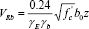Figure 74. Equation. Shear capacity as a function of concrete strength.

The fiber contribution, Vf, within the AFGC recommended provisions is defined by the equation in figure 75. The variable S is the area of the shear plane being considered. This variable is assumed to equal 90 percent of the web width multiplied by the depth to the centroid of the prestressing strands. bu is the angle of the compression struts in the shear area as measured from the horizontal. The assumption that the angle is 40° is a conservative estimate of the angle based on the prestressed concrete girder behavior. The variable gbf defines a factor of safety that is set equal to 1.3. Finally, sp defines the average residual tensile stress carried by the fibers across a shear crack from cracking until a limiting strain value is achieved. This value is normally based on experimental tension test results. In this case, based on the results presented in section 6.4 and the associated material characterization report, the residual tensile stress will be assumed to be 6.9 MPa (1.0 ksi).(2) Thus, the design value for the fiber contribution to the UHPC shear capacity is 695 kN (156 kips). Alternatively, setting the factor of safety terms again to 1.0 results in a fiber contribution to the shear capacity of 760 kN (170 kips).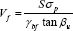Figure 75. Equation. Fiber contribution to shear strength.

These girders contain no mild steel reinforcement and the prestressing strands are not draped; therefore, the concrete and fiber contributions are the only two items relevant to determining the shear capacity. In total, the AFGC recommendations indicate that the ultimate design shear capacity of the AASHTO Type II prestressed girders tested in this study is 921 kN (207 kips). Eliminating the factor of safety terms produces the true AFGC estimate of the shear capacity, which is 1,100 kN (247 kips). Although this method of estimating the shear capacity of UHPC girders is more rigorous than many shear capacity calculations, the method still clearly significantly underestimates the shear capacity of these UHPC girders.

### 6.5.2 Cracking Behavior

The shear cracking of the UHPC girders during the shear tests is again indicative of the structural cracking behaviors associated with UHPC. First, this section discusses the cracking behaviors observed during the shear tests. Then the cracking behavior of Girder 24S is analyzed to determine the effective prestress force applied to the girder.

Girders 24S and 14S both exhibited diagonal web shear cracking that eventually led to the failure of the girders. Girder 24S first exhibited shear cracking at a shear load of 1,130 kN (254 kips). The cracking initiated in the portion of the shear span closer to the load point, because this location experienced both high shear stresses and larger flexural forces. The angle of the shear cracks at this location was approximately 35° from the horizontal. Girder 14S first exhibited shear cracking at a shear load of 912 kN (205 kips). Again, shear cracking initiated in the web close to the load point with an angle of 38° from the horizontal.

The number of shear cracks in both girders increased as the shear load increased. Initially, the cracks were spaced approximately 25 mm (1 inch) apart throughout the web from the load point to the bearing. As the test progressed, the crack spacing decreased as more cracks appeared until the crack spacing had decreased to 6 mm (0.25 inch) or less in some locations.

As presented in chapter 4, Girder 24S failed when the fibers bridging a highly stressed shear crack began to pull out. In this girder, the loss of tensile capacity across the shear crack resulted in immediate failure because the shear forces could not be redistributed around the crack. The cracking and eventual fiber pullout across a crack in Girder 14S resulted in a different type of failure. In this girder, the shear capacity and girder stiffness decreased simultaneously as the fibers pulled out and the strands slipped, thus allowing for a slow decrease in shear capacity until more than one-fourth of the shear load had been shed.

The first cracking behavior of a prestressed girder is indicative of both the tensile cracking strength and the level of prestress applied to the girder. The method used to test Girder 24S resulted in the prestressing strands fully transferring their stress into the girder outside of the highly stressed shear region. The analysis of this girder enabled the verification of the prestress force and tensile cracking strength values that were discussed previously. Shear and flexural strains at cracking on an element in the web of this girder located under the rosette strain gage closest to the load point indicate that 161 microstrain of tension caused cracking. If the tensile cracking strain of UHPC is assumed to be 200 microstrain, the effective prestress force on this girder would be 2,025 kN (455 kips), which was determined in section 6.3. Note that a 200-microstrain tensile cracking strain would correspond to a 55.2-GPa (8000-ksi) modulus of elasticity with linear elastic behavior through cracking at 11 MPa (1.6 ksi). This tensile cracking behavior is consistent with the behaviors discussed in the associated UHPC material characterization report.(2)

### 6.5.3 Simplified Model of UHPC Girder Shear Failure

The girder shear behaviors observed during the testing of Girders 24S and 14S indicate that the shear behavior of a UHPC I-girder containing no mild steel shear reinforcement is less complex than is normally associated with a concrete girder. For example, determination of the shear capacity of a concrete girder could involve the estimation of the capacity provided by the concrete alone, the capacity provided by the mild steel reinforcement alone, and the capacity that results from the interaction of the mild steel reinforcement to enhance the concrete capacity. The terms associated with concrete behavior are normally difficult to ascertain and frequently are approximated through empirical relationships. Observations from the UHPC girder tests indicate that the shear behaviors of a UHPC girder as failure is approached are significantly less complicated and lend themselves to basic modeling and approximation procedures.

The descriptions of the failure of Girder 24S, in particular the photographs shown in figure 38, clearly illustrate that the failure of this girder was caused by diagonal tensile forces in the highly stressed shear region of the girder. Because this UHPC girder contained no mild steel, this tensile failure of the concrete was not influenced by reinforcing bars bridging the cracks. Also, because UHPC has a very fine granular structure, it is not anticipated that any significant level of aggregate interlock could be present in a UHPC girder. All indications-including the observations of many tightly spaced cracks parallel to the eventual tensile failure plane-lead to the conclusion that simply determining the vertical component of the tensile stress capacity over the expected shear failure plane would provide an indication of the shear capacity of the girder.

This simple analytical procedure is conceptually equivalent to the fiber contribution to shear capacity (detailed in the AFGC recommended provisions and discussed in section 6.5.1). In the AFGC design procedure, the fiber contribution to the shear capacity is composed of terms representing the average tensile capacity of UHPC at failure, the angle of the failure plane relative to the girder, and a partial depth of the cross section. Algebraic manipulation shows that the AFGC equation actually estimates the shear failure plane area, multiplies it by the average estimated tensile stress over the failure plane, and converts the result into a vertical component that is useful for shear calculations.

Thus, the knowledge of two girder-specific properties and one material property allows for the estimation of the shear capacity of the girder. During design, the angle of the failure plane can be estimated based on the level of prestress in the girder. The area of the failure plane is more difficult to estimate. However, it should be able to be bounded based on the thickness of the web, the height of the web and web transitions, and the angle of the failure plane. Finally, the average tensile stress exhibited by UHPC prior to fiber pullout must be estimated based on prior knowledge of the material behavior.

Test results from Girders 24S and 14S can be used to illustrate the capabilities of this simple analytical procedure. The failure plane angles in these two girders were measured to be 28° and 25° from the horizontal, respectively. The peak shear loads carried by these girders before the fiber pullout were 2,225 and 1,950 kN (500 and 438 kips), respectively. The depth of the cross section that the failure plane traverses can be estimated to be between the depth of the web, 0.38 m (15 inches), and the AFGC recommended depth, 0.71 m (28 inches). Observed shear cracking behaviors during the tests indicate that most diagonal tensile shear loads were carried by the girders within the web and a small part of the web transitions. Thus, for this analysis the depth of the failure plane is assumed to be 0.48 m (19 inches). By assuming this depth of failure plane over the width of the web at the failure plane angles mentioned above, the geometry of the failure surface has been defined. Using these values, the average tensile stress in Girder 24S is determined to be 15.9 MPa (2.3 ksi). In Girder 14S the value is determined to be 12.4 MPa (1.8 ksi).

These average tensile stress values before fiber pullout are reasonable approximations of UHPC behavior based on results presented elsewhere in this report. The tensile stress-strain behavior derived in section 6.4.5 and presented in figure 70 is of greater relevance for this discussion. In that analysis of the flexural loading of a prestressed girder, the figure shows that the average tensile stress prior to fiber pullout was more than 17.2 MPa (2.5 ksi). Because the shear regions in Girders 24S and 14S were not supplementally reinforced by prestressing strands like the bottom flange of a prestressed girder, it is understandable that the average stress across the failure plane might be lower prior to fiber pullout. Regardless, this analytical procedure clearly provides a reasonable interpretation of shear failure behavior based on sound engineering principles without reverting to empirical relationships.

### 6.5.4 Strut-and-Tie Model of Girder 28S Failure

The fiber-reinforced concrete matrix is capable of carrying and dispersing both tensile and compressive loads. Therefore, in general, truss modeling of UHPC behavior is likely to be less useful than truss modeling on normal or HPC behavior. Thus, the tension ties that are a major part of most truss models become significantly less relevant with UHPC.

However, the final failure of Girder 28S involved crushing of the web concrete and tensile rupture of the top flange. These behaviors were not expected in a girder shear test, which leads to the development of a truss model to explain the global behavior at failure. Recall that this girder test included the development of a shearing plane along the base of the web in the highly stressed shear region.

Figure 76 displays the truss system that was developed to model the failure of Girder 28S. In this figure, the brown dashed lines indicate compression struts while the solid green lines indicate tension ties. The design of this model includes a complete delamination of the web from the bottom flange within the highly stressed shear region, which ensures that no forces were able to transfer across this boundary.

At failure, the applied load on this girder was 2,225 kN (500 kips), with 1,710 kN (384 kips) being carried by the east support. Given this loading, the forces in kN in the compression struts of the truss model include: A = -1,109, B = -1,598, C = -338, D = -1,455, E = -1,046, H = -1,041, J = -2,092, K = -156, P = -3,493, Q = -2,407, S = -1,486, and T = -3,582. The forces in the tension ties include: F = 1,068, G = 1,099, I = 961, L = 1,210, and N = 2,861.

Although truss models provide an upper bound to the solution, an examination of this answer indicates that it is a reasonable solution. In particular, a detailed analysis shows that the node connecting seven compression struts just above the bearing is the critical node. As was observed in the girder test, the compression forces shown in the struts would create a compression failure of this 193-MPa (28-ksi) UHPC at this node. This model also reasonably predicts the subsequent tensile failure of the top flange above the bearing. Tension tie L shown in figure 76 carries 1,210 kN (272 kips). This load is large enough to cause tensile cracking of the UHPC top flange and to initiate fiber pullout leading to strain localization in the strands and strand rupture.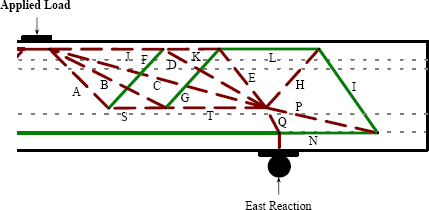Figure 76. Illustration. Truss model for failure of Girder 28S.